search for books and compare prices
Ernst Hairer has written 8 work(s)
Search for other authors with the same name
displaying 1 to 8 | at end
show results in order: alphabetically | oldest to newest | newest to oldest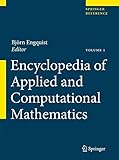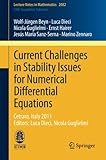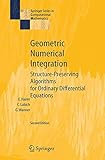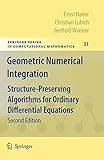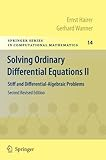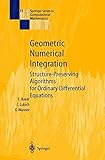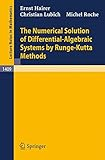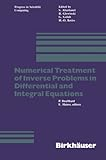Product Description: EACM is a comprehensive reference work covering the vast field of applied and computational mathematics. Applied mathematics itself accounts for at least 60 per cent of mathematics, and the emphasis on computation reflects the current and constantly growing importance of computational methods in all areas of applications...read more
By Tony Chan (editor), William J. Cook (editor), Bjârn Engquist (editor), Ernst Hairer (editor) and Johan Hastad (editor)

Hardcover:

9783540705307 | Har/psc edition (Springer Verlag, November 23, 2015), cover price \$1560.00 | About this edition: EACM is a comprehensive reference work covering the vast field of applied and computational mathematics.

Product Description: This volume addresses some of the research areas in the general field of stability studies for differential equations, with emphasis on issues of concern for numerical studies. Topics considered include: (i) the long time integration of Hamiltonian Ordinary DEs and highly oscillatory systems, (ii) connection between stochastic DEs and geometric integration using the Markov chain Monte Carlo method, (iii) computation of dynamic patterns in evolutionary partial DEs, (iv) decomposition of matrices depending on parameters and localization of singularities, and (v) uniform stability analysis for time dependent linear initial value problems of ODEs...read more

Paperback:

9783319012995 | Springer Verlag, December 18, 2013, cover price \$59.99 | About this edition: This volume addresses some of the research areas in the general field of stability studies for differential equations, with emphasis on issues of concern for numerical studies.

Product Description: This book covers numerical methods that preserve properties of Hamiltonian systems, reversible systems, differential equations on manifolds and problems with highly oscillatory solutions.

Hardcover:

9783540306634 | 2 edition (Springer Verlag, May 16, 2006), cover price \$199.00 | About this edition: This book covers numerical methods that preserve properties of Hamiltonian systems, reversible systems, differential equations on manifolds and problems with highly oscillatory solutions.

Paperback:

9783642051579 | 2 edition (Springer Verlag, January 15, 2010), cover price \$119.00 | About this edition: This book covers numerical methods that preserve properties of Hamiltonian systems, reversible systems, differential equations on manifolds and problems with highly oscillatory solutions.

Product Description: The subject of this book is the solution of stiff differential equations and of differential-algebraic systems. This second edition contains new material including new numerical tests, recent progress in numerical differential-algebraic equations, and improved FORTRAN codes...read more

Paperback:

9783642052200 | 2 revised edition (Springer Verlag, January 15, 2010), cover price \$119.00 | About this edition: The subject of this book is the solution of stiff differential equations and of differential-algebraic systems.

Product Description: Numerical methods that preserve properties of Hamiltonian systems, reversible systems, differential equations on manifolds and problems with highly oscillatory solutions are the subject of this book. A complete self-contained theory of symplectic and symmetric methods, which include Runge-Kutta, composition, splitting, multistep and various specially designed integrators, is presented and their construction and practical merits are discussed...read more (view table of contents, read Amazon.com's description)

Hardcover:

9783540430032 | Springer Verlag, June 1, 2002, cover price \$107.00 | About this edition: Numerical methods that preserve properties of Hamiltonian systems, reversible systems, differential equations on manifolds and problems with highly oscillatory solutions are the subject of this book.

Product Description: The term differential-algebraic equation was coined to comprise differential equations with constraints (differential equations on manifolds) and singular implicit differential equations. Such problems arise in a variety of applications, e...read more

Paperback:

9780387518602 | Springer Verlag, December 1, 1989, cover price \$17.50 | About this edition: The term differential-algebraic equation was coined to comprise differential equations with constraints (differential equations on manifolds) and singular implicit differential equations.
9783540518600 | Springer Verlag, November 28, 1989, cover price \$39.99 | About this edition: The term differential-algebraic equation was coined to comprise differential equations with constraints (differential equations on manifolds) and singular implicit differential equations.

Product Description: In many scientific or engineering applications, where ordinary differen­ tial equation (OOE),partial differential equation (POE), or integral equation (IE) models are involved, numerical simulation is in common use for prediction, monitoring, or control purposes...read more

Paperback:

9780817631253 | Birkhauser, May 1, 1983, cover price \$99.00 | About this edition: In many scientific or engineering applications, where ordinary differen­ tial equation (OOE),partial differential equation (POE), or integral equation (IE) models are involved, numerical simulation is in common use for prediction, monitoring, or control purposes.

displaying 1 to 8 | at end